Next: Inversion Up: The Sphere in Three Previous: Overview of the Sphere

Subsections

# Visualizing the N-Sphere

## The two balls model

This observation about equatorial surfaces can be used as an aid to visualization as well.

### The two balls model of
First, imagine that we were beings who inhabited a two-dimensional universe. Visualizing three-dimensional space would (probably) not come naturally to us, and thus neither would visualizing, which needs to be embedded in at least three dimensions.

However, we could visualizeby thinking of it as two disks, plus a special rule. The special rule goes like this: whenever you cross the boundary of one disk, you reappear at the corresponding point on the boundary of the other disk. Each disk is a hemisphere, and the boundary is the equator. Thought of this way, the rule becomes obvious: whenever you leave one hemisphere, and cross the equator, you reappear at the corresponding point in the other hemisphere.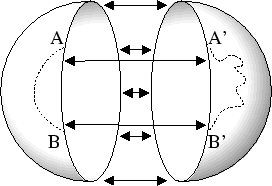Note that when our two dimensional being lays the two disks flat out in
, there are two ways that they can be laid down. First, both disks can simply be flattened onto. If this is done, then a vector pointing out of the sphere on the lower hemisphere will be pointing down from, and a vector pointing out of the sphere on the upper hemisphere will be pointing up on. Alternately, the bottom hemisphere can be reflected or flipped, so that a vector pointing out on the sphere will be pointing up from, on both hemispheres. Note that this act of flipping means that we will have to be extra careful about how we identify the edges of the hemisphere; specifically, points will be mapped to the corresponding point on the hemisphere after the reflection. For practical purposes, we will consider the first model where the lower hemisphere is not flipped.

### The two balls model of
Now, imagine that we are beings who inhabit a three dimensional universe (Hopefully this is not too hard). Visualizing four-dimensional space would not come naturally to us, and neither would visualizing, which needs to be embedded in at least four dimensions. But we can visualize the hypersphere by thinking of it as two filled balls, or filleds, plus a special rule, just like in the two dimensional case.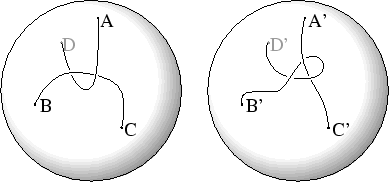Finally, similarly to the two disks model of, we can flatten the hemispheres ontowithout flipping one, or with flipping one. Just as before, we will use the model without the flip.

### Using a two balls model to visualize higher dimensional spheres

s If we wanted to visualize
, we could first visualizein this way, and then make two copies of, and consider each to be a
or filled. We could then glue each point in theto a corresponding point in the other, and we would have. Unfortunately, this becomes a bit cumbersome and complicated for dimensions higher than three.

## Stereographic Projection

Stereographic projection is a way of projecting
onto
. Intuitively, it can be thought of as the process of puncturing the sphere and stretching it out flat, onto Euclidean space. It too can be used as an aid to visualization.

### Stereographic Projection of
To stereographically projectonto
, first choose an equator, and a north pole.'' In the case of, the equator simply is two antipodal points on the circle, and the north pole is the point on the circle equally between them. For simplicity, let's assume the circle is the unit circle, the north pole is the point (0,1), and the equator the set of points
. We are now going to map, or stereographically project, the circle onto the x axis.

The map is as follows: Draw a line from the north pole, through a point
on the circle, and extend it until it hits the x-axis at the point
. Stereographic projection is simply the map which takes each pointon the circle and maps it to the pointin. In the case with, the map sends
to
.

Notice that every point onhas an image on, except for the north pole itself. The north pole is considered to be mapped to a point at infinity. So we can say that stereographic projection from
is a bijection.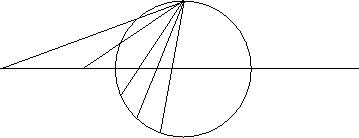As mentioned above, stereographic projection can be thought of as puncturing the sphere, and stretching it out flat onto the correct Euclidean space. Points in the southern hemisphere'' are mapped to points between 1 and
, and points in the northern hemisphere'' are mapped to points outside.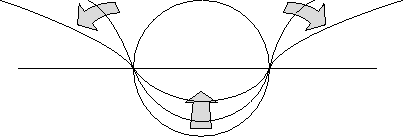### Stereographic Projection ofTo stereographically project,imagine, centered at the origin. To project the sphere onto the x-y plane, we choose the north pole'' to be the point
. Then, to project a point
onto the x-y plane, we draw a line from the north pole, through
to it's image point
on the x-y plane.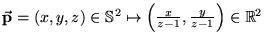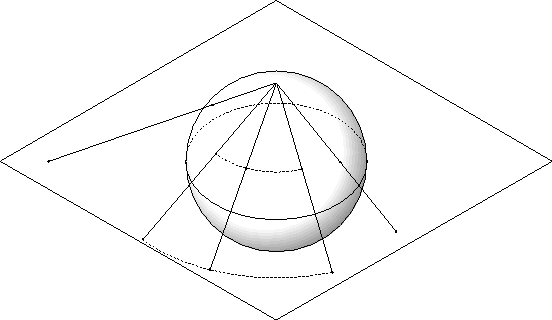Again, the north pole is mapped to the point at infinity. Now the hypothetical two-dimensional beings have another way to visualize: they can think of it as regular, plus a point at infinity. If they were moving, say, a little square around the sphere, and watching how it's image changed in the plane as it moved, they would see it get larger as it got further from the south pole, which is mapped to the the origin on the plane. If they moved the square from the south pole, through the north pole, and back to the south pole, the two dimensional beings would see the square get larger and larger as it approached the north pole. When the north pole was inside the square, they would see the square all around them. As the square moved back to the south pole, they would see the square approaching from the other direction, getting smaller as it approached.

### Stereographic Projection inStereographic projection is possible in general, for. The map is: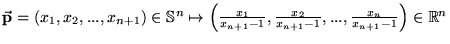Now we have another way to visualize the three sphere: we can think of it as
, plus a point at infinity. If we were moving a cube around the three sphere, and looking at it's image in Euclidean three-space under stereographic projection, it would get bigger as it got further from the south pole, which is the origin in. Like the image ofin, if we were moving a cube along a great circle from the south pole, through the north pole, back to the south pole, we would see it start out small at the origin, get larger as it went further from the origin. When the north pole crossed inside the little cube, we would see the cube all around us. Then, as the square passed back towards the south pole, we would see it shrink again as it approached.

Stereographic projection offers another technique of visualizing or thinking about higher dimensional spheres, and another point of view on.Next: Inversion Up: The Sphere in Three Previous: Overview of the Sphere